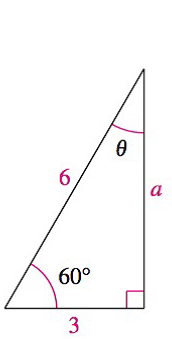Chapter 8.1, Problem 35E### Calculus: An Applied Approach (Min...

10th Edition
Ron Larson
ISBN: 9781305860919

#### Solutions

Chapter
Section### Calculus: An Applied Approach (Min...

10th Edition
Ron Larson
ISBN: 9781305860919
Textbook Problem
1 views

# Analyzing Triangles In Exercises 33-40, solve the triangle for the indicated side and/or angle.To determine

To calculate: The value of side a and angle θ in a triangle provided below.Explanation

Given Information:

The provided triangle is shown below,

Formula used:

In a right triangle, the sum of two acute angles is 90°.

Pythagorean theorem for any right triangle is such that c2=a2+b2, where a, b are the two perpendicular sides of a triangle and c is the length of hypotenuse.

Calculation:

Consider the sides of triangle, 6, 3 and a

The value of side b is 3 and hypotenuse c is 6.

Substitute b=3 and c=6 in the equation a2+b2=c2

### Still sussing out bartleby?

Check out a sample textbook solution.

See a sample solution

#### The Solution to Your Study Problems

Bartleby provides explanations to thousands of textbook problems written by our experts, many with advanced degrees!

Get Started

#### Expand each expression in Exercises 122. (xx2)(x+x2)

Finite Mathematics and Applied Calculus (MindTap Course List)

#### In problems 45-62, perform the indicated operations and simplify. 59.

Mathematical Applications for the Management, Life, and Social Sciences

#### True or False: is a geometric series.

Study Guide for Stewart's Single Variable Calculus: Early Transcendentals, 8th